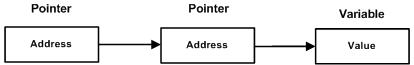# Pascal - Pointer to Pointer

A pointer to a pointer is a form of multiple indirection or a chain of pointers. Normally, a pointer contains the address of a variable. When we define a pointer to a pointer, the first pointer contains the address of the second pointer, which points to the location that contains the actual value as shown below.A variable that is a pointer to a pointer must be declared as such. For example,

```type
iptr = ^integer;
pointerptr = ^ iptr;
```

Following example would illustrate the concept as well as display the addresses −

```program exPointertoPointers;
type
iptr = ^integer;
pointerptr = ^ iptr;

var
num: integer;
ptr: iptr;
pptr: pointerptr;
x, y : ^word;

begin
num := 3000;

(* take the address of var *)
ptr := @num;

(* take the address of ptr using address of operator @ *)
pptr := @ptr;

(* let us see the value and the adresses *)

writeln('Value of num = ', num );
writeln('Value available at ptr^ = ', ptr^ );
writeln('Value available at pptr^^ = ', pptr^^);
writeln('Address at ptr = ', x^);
writeln('Address at pptr = ', y^);
end.
```

When the above code is compiled and executed, it produces the following result −

```Value of num = 3000
Value available at ptr^ = 3000
Value available at pptr^^ = 3000